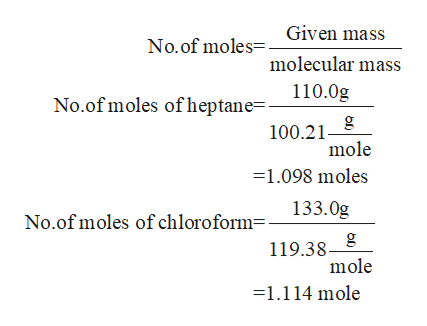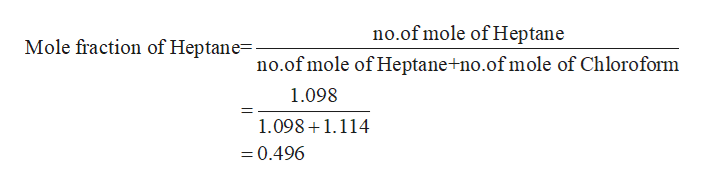# Using Raoult's Law to calculate the vapor pressure of a compon...DEAt a certain temperature the vapor pressure of pure heptane 716and 133. g of chloroform (CHCI)is measured to be 0.50 atm. Suppose a solution is prepared by mixing 110. g of heptaneCalculate the partial pressure of heptane vapor above this solution. Round your answer to 2 significant digits.Note for advanced students: you may assume the solution is ideal.atmX10?CheckExplanationFTerms of Use2019 McGraw-Hill Education. All Rights Reserved.

Question
1 views

Calculate the partial pressure of heptane vapor above this solution. Round to two sig figs

check_circle

Step 1

Given that,

Vapor pressure of pure heptane = 0.50 atm

Mass of heptane = 110.0 g

Mass of Chloroform = 133.0 g

Step 2

Now, you calculate the no.of mole of heptanehelp_outlineImage TranscriptioncloseGiven mass No.of moles= molecular mass 110.0g No.of moles of heptane=- 100.21 mole =1.098 moles 133.0g No.of moles of chloroform- 119.38 mole =1.114 mole fullscreen
Step 3

Now, you calculate the mole...help_outlineImage Transcriptioncloseno.of mole of Heptane Mole fraction of Heptane- no.of mole of Heptane no.of mole of Chloroform 1.098 1.0981.114 =0.496 fullscreen

### Want to see the full answer?

See Solution

#### Want to see this answer and more?

Solutions are written by subject experts who are available 24/7. Questions are typically answered within 1 hour.*

See Solution
*Response times may vary by subject and question.
Tagged in

### Chemistry